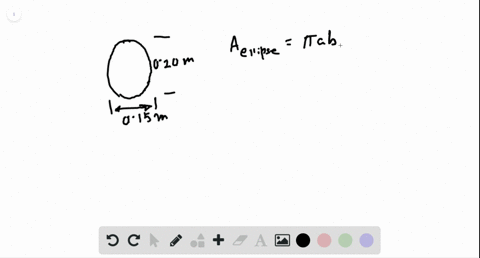🎉 The Study-to-Win Winning Ticket number has been announced! Go to your Tickets dashboard to see if you won! 🎉View Winning Ticket### Problem 11

03:36H.B.T.I.
Problem 10

# How do you position a bicycle tire so that the magnetic flux through it due to Earth's magnetic field is as large as possible? Estimate this maximum flux. What assumptions did you make?

## Discussion

You must be signed in to discuss.

## Video Transcript

Hi. In the given problem, suppose this is arts total magnetic field in the human region. Then it will be having its two components. One of the component is horizontal edge, which is equal to because Delta here, this delta is angle of inclination off this magnetic field and another will be vertical component which is given by B sign Delta No, we have toe arrange a bicycle tire in this magnetic field in such a way that the maximum magnetic flux may pass through it. So the only way toe arrange this, Mac, this bicycle tire is toe put it just perpendicular toe Earth's total magnetic field. Hence we can say the tire should be gift such that the area victor off this tire is parallel to magnetic field vector. So this is magnetic field vector here. The area vector should also be lying along it. So this is a bar over here, and that is be bar over here. Hence the flux Passing through this coil will become passing through. This tire will become b dot A. Or we can say this is be a cause zero degree and we know the value. Of course. Science zero degree is maximum, which is one hence, the maximum magnetic flux. Passing through this tire is given us the product off B is the magnetic field in that region with the area off this tire. Yeah, our resumption is that the diameter, the average diameter off bicycle tire is around 800 millimeter soaked. It's radius will be 400 millimeter. Or we can say this is 0.4 meter and the magnetic field in that region may be assumed. Toby, this is also our assumption. This magnetic will maybe taken us five 0.8 approximately into 10 dish bar minus five slop. Hence this magnetic flux. This maximum magnetic flux passing through this bicycle tire is given as the product of B into A for B, this is 5.8 in tow. 10 dish par minus five. Tesla for area. This is by our square and we know the value off previous. This is the square off 0.4 meter square and then we calculated the maximum flux passing through the bicycle tire comes out to be 2.91 in tow. 10 raised the bar minus five Tesler meters squared. Or we can say this is 2.91 in tow. 10 dish par, minus five V ever. So this is the answer. Forgiven problem. Thank you.

#### You're viewing a duplicate answer. The book and chapter may be different but the question is the same.

To request the exact answer, fill out the form below

Our educator team will work on creating an answer for you in the next 6 hours.